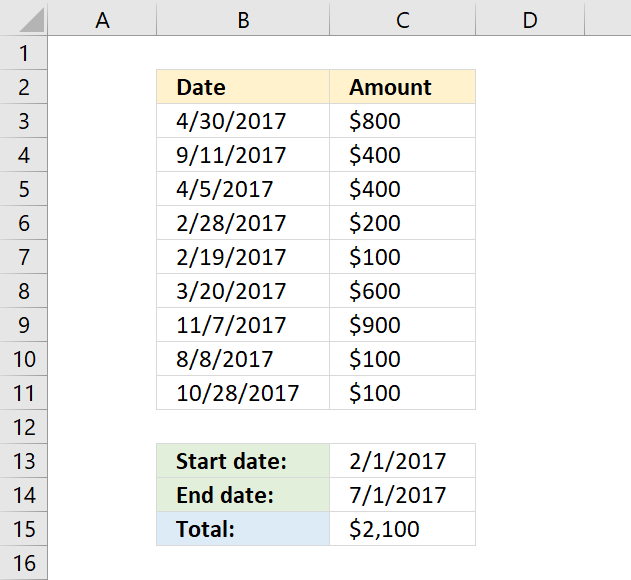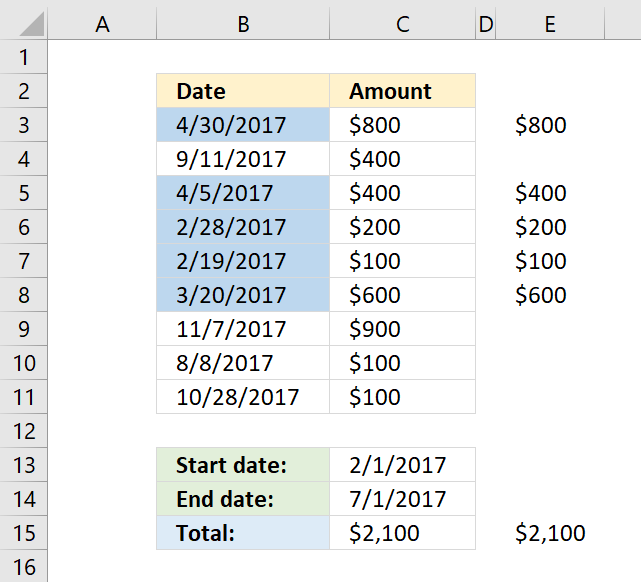Author: Oscar Cronquist Article last updated on November 14, 2018The formula in cell C15 uses two dates two to filter and then sum values in column C, the SUMIFS function is designed to use multiple conditions.

=SUMIFS(C3:C11,B3:B11,"<="&C14,B3:B11,">="&C13)

The first condition filters dates less than or equal to the end date in cell C14.

"<="&C14

The second condition filters dates larger than or equal to the start date in cell C13.

">="&C13

The ampersand character lets you concatenate the logical operators to the date value. The following picture shows the date values that match both conditions.The SUMIFS function has the following arguments:

SUMIFS(sum_range, criteria_range1, criteria1, [criteria_range2, criteria2], ...)

### Sum by date range

The SUMIFS was introduced in Excel 2007, the following formula works with Excel versions earlier than 2007.

=SUMPRODUCT(C3:C11*(B3:B11<=C14)*(B3:B11>=C13))

### Get excel *.xlsx

Sum between two dates.xlsx